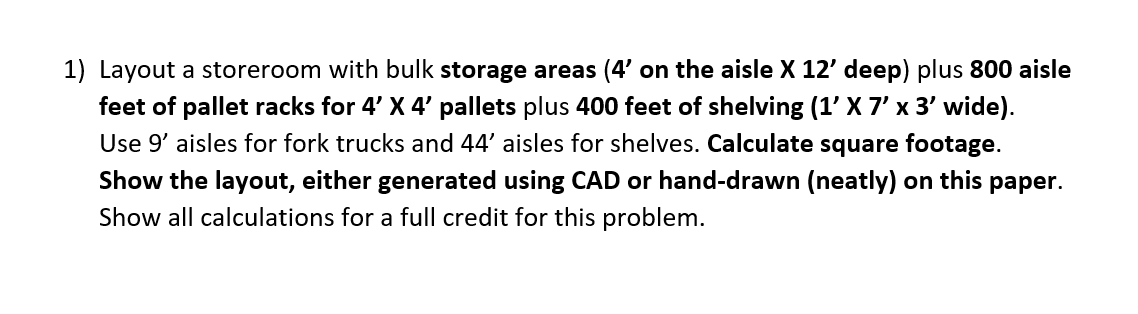# Question 1) Layout a storeroom with bulk storage areas (4' on the aisle $$X 12^{\prime}$$ deep) plus 800 aisle feet of pallet racks for $$4^{\prime} \times 4^{\prime}$$ pallets plus 400 feet of shelving ( $$1^{\prime} \times 7^{\prime} \times 3^{\prime}$$ wide). Use $$9^{\prime}$$ aisles for fork trucks and 44' aisles for shelves. Calculate square footage. Show the layout, either generated using CAD or hand-drawn (neatly) on this paper. Show all calculations for a full credit for this problem.Transcribed Image Text: 1) Layout a storeroom with bulk storage areas (4' on the aisle $$X 12^{\prime}$$ deep) plus 800 aisle feet of pallet racks for $$4^{\prime} \times 4^{\prime}$$ pallets plus 400 feet of shelving ( $$1^{\prime} \times 7^{\prime} \times 3^{\prime}$$ wide). Use $$9^{\prime}$$ aisles for fork trucks and 44' aisles for shelves. Calculate square footage. Show the layout, either generated using CAD or hand-drawn (neatly) on this paper. Show all calculations for a full credit for this problem.
Transcribed Image Text: 1) Layout a storeroom with bulk storage areas (4' on the aisle $$X 12^{\prime}$$ deep) plus 800 aisle feet of pallet racks for $$4^{\prime} \times 4^{\prime}$$ pallets plus 400 feet of shelving ( $$1^{\prime} \times 7^{\prime} \times 3^{\prime}$$ wide). Use $$9^{\prime}$$ aisles for fork trucks and 44' aisles for shelves. Calculate square footage. Show the layout, either generated using CAD or hand-drawn (neatly) on this paper. Show all calculations for a full credit for this problem.